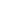Flat 50% off

Ends in

• NEET
•• 11th
•• Physics
• Back to Doubt Clearing

## 1.Assertion:The position time graph of a body moving uniformly in a straight line parallel to position axis. Reason:The position time graph in a uniform motion gives the velocity of an object.2.Assertion:Average speed is equal to total distance travelled divided by total time taken. Reason:The average speed of an object may be equal to arithmetic mean of individual speed. MY DOUPT: AVERAGE SPEED = $\frac {(V_1+V_2)}2$, FOR FIRST HALF OF TIME , the velocity is $V_1$ and the NEXT HALF OF TIME, the velocity is $V_2$.Form this situiation average speed may be equal to arithmetic mean of individual speed.

### Asked By Sivakumar.S

Updated Fri, 28 Sep 2018 12:57 pm